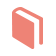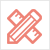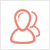﻿ 更多范文-Assignment代写：Homework Assignment-51Due留学教育

#### 服务承诺资金托管原创保证实力保障24小时客服使命必达

#### 关于我们

51Due提供Essay，Paper，Report，Assignment等学科作业的代写与辅导，同时涵盖Personal Statement，转学申请等留学文书代写。51Due将让你达成学业目标51Due将让你达成学业目标51Due将让你达成学业目标51Due将让你达成学业目标

#### 名企实习积累工作经验多元化文化交流专业实操技能建立人际资源圈

# Assignment代写：Homework Assignment

2018-04-17 来源: 51due教员组 类别: 更多范文

Due: Noon, 27th Nov, 2017

Submit printed solutions via eClass

Submit handwritten solutions at dropbox on CSC level 1

CMPUT 204

Department of Computing Science University of Alberta

Note: All logs are base-2 unless stated otherwise.

You should answer at least 3 out of Problems 1-4, and must answer Problem 5. Should you answer all of the first 4 problems, your grade will be composed of the best 3 out of 4.

Exercise I. Let G be a graph whose vertices are the integers 1 through 8, and let the adjacent vertices of each vertex be given by the table below:

vertex 1

2

3

4

5

6

7

8

adjacent vertices {2, 3, 4}

{1, 3, 4}

{1, 2, 4} {1,2,3,6} {6, 7, 8}

{4, 5, 7}

{5, 6, 8} {5,7}

(a) Draw G.

(b) Write G in the adjacency matrix model.

(c) Order the vertices as they are visited in a BFS traversal starting at vertex 1. Draw the BFS tree.

(d) Order the vertices as they are visited in a DFS traversal starting at vertex 1. Draw the DFS tree. Suggestion. If you are having issues with understanding the way BFS/DFS operate, repeat this question with other graphs!

Problem 1. (20 pts) Given a graph G (directed or undirected) it’s 2-closure is the graph H such that V (H) = V (G) and for any two nodes u, v ∈ V (H) we put an edge if in G we have that v is reachable from u by a path of length 1 or 2. (Therefore, it is clear that any e ∈ E(G) will remain an edge also in E(H).)

(a) (10 pts) Give a deterministic algorithm that takes as an input a graph G with max-degree of ∆ in the adjacency list model and outputs its 2-closure in O(n∆2)-time.

Note: our construction of a Hash-Table, for example, is inherently random.

(b) (10 pts) Give an algorithm that takes as an input a graph G in the adjacency matrix model and outputs its 2-closure in o(n3)-time.

Hint: We call it an adjacency matrix for a reason…

Problem 2. (20 pts) A vertex s of a directed graph G(V, E) is called a sink if for every vertex v ̸= s, it holds that (v, s) ∈ E and (s, v) ̸∈ E. In other words, every vertex has an edge to s and no edges leave s. The graph is given by an adjacency matrix A.

Give an algorithm takes as an input a directed graph G and either finds a sink or returns “no sinks” in only O(n) time. Argue that your code is correct.

Notice that a running time of O(n) is remarkable given that the input can have potentially O(n2) edges. Hint: It is enough for your algorithm to find a single candidate for being a sink, then verify whether it is indeed a sink or not. Make sure your algorithm runs in O(1) time per candidate elimination.

1

Problem 3. (20 pts) Give an algorithm that solves mazes. The maze is a rectangular maze with r rows and c columns, given in the form of a function IsWall(i, j, D) that tells you, in O(1)-time, whether you can or cannot move from square (i, j) in direction D (where D ∈ {Up, Down, Left, Right}). You are also given a start position s = (i,j) and a finish position f = (i′,j′). Your goal is to find the shortest path taking you from s to f (if such a path exists). Your algorithm must run in O(r · c) time.

Explain why your algorithm is correct and why its runtime is O(r · c).

Figure 1:

Problem 4. (20 pts) Recall how we increase the capacity of a stack (or a queue, or a hash-table) once a

A rectangular maze.

Push() call is invoked on a full stack — using IncreaseCapacity():

1. Allocate a new array with 2 × stack.capacity cells

2. Copy all items from the original array to the new array, delete the old array

Analogously to this, we can decrease capacity and clear some memory once stack.size is small in comparison to its stack.capacity — using DecreaseCapacity():

1. Allocate a new array with stack.capacity/2 cells

2. Copy all items from the original array to the new array, delete the old array Note that both IncreaseCapacity() and DecreaseCapacity() run in Θ(n)-time.

(a) (5 pts) Show that invoking DecreaseCapacity() whenever the number of elements in the stack (i.e., stack.size) is stack.capacity/2 is unwise. That is, show that there exists a sequence of n Push() / Pop() instructions whose amortized cost is Ω(n).

(b) (15 pts) Prove that invoking DecreaseCapacity() whenever stack.size = stack.capacity/3 maintains constant amortized cost. That is, show that any sequence of n Push() / Pop() instructions has amortized cost of O(1). You may assume the initial Stack capacity is 1.

Here is a suggested outline:

Call an instruction heavy if it invokes either IncreaseCapacity() or DecreaseCapacity(); and call it light otherwise. Light instructions always take O(1). Your argument should probably begin by showing that between any two heavy instructions there have to be many light instructions (by considering all 4 possible cases), where m denotes the capacity of the stack between the two heavy instructions. Then use this claim to prove, by induction, that T (n) — defined as the worst-case cost of a sequence n Push()/PoP() instructions — is in O(n).

2

Problem 5. (40 pts) Given a connected undirected graph G = (V, E) on n nodes and m ≥ n − 1 edges, we introduce the following definitions.

Figure 2: The articulation points (heavily shaded vertices), and biconnected components (shaded regions with numberings) in a toy example.

An articulartion point is a node v such that the removal of v and its adjacent edges leaves G uncon- nected.

A bridge is an edge e = (u, v) such that the removal of e leaves G unconnected.

Biconnected pair of vertices u, v ∈ V (G) is a pair of vertices with two vertex-disjoint paths from u to v.

In other words, there’s a simple cycle containing both u and v.

A biconnected component is a maximal set of edges such that each pair of edges lie on some simple

cycle.

In this question, we show how DFS finds all vertices / edges which satisfy the above definitions.

(a) (3 pts) In the DFS-tree of G, prove that the root is an articulation point if and only if its has more than one child.

(b) (9 pts) In the DFS-tree of G, fix a non-root node v and its child u. We say u is v-bypassing if there exists a back edge connecting either u or a descendant of u with an ancestor of v (an ancestor that cannot be v itself). We say v is a fully-passed node is all of its children are v-bypassing.

Prove that a non-root v is an articulation point if and only if it is not a fully-passed node.

(c) (1 pts) Given the DFS tree rooted at a node s, we define for each node v the attribute v.dist =distance between s and v on the DFS-tree. Argue that A BFS traversal on the DFS-tree assigns each node its v.dist attribute in O(n) time. (Note the runtime is independent of the number of edges in G.)

(d) (7 pts) We also define

v.l = min {v.dist; w.dist for some back-edge connecting a descendant u of v with some w }

Show how to find v.l for all vertices in G in O(n + m) time. Argue the correctness and runtime of your algorithm.

(e) (5 pts) Give an algorithm that finds all articulation points of G in O(n + m) time. Argue your algorithm correctness and runtime.

(f) (5 pts) Prove that an edge e = (u,v) is a bridge iff it doesn’t lie on any simple cycle in G.

(g) (5 pts) Give a O(n + m) algorithm for finding all bridges in G.

(h) (5 pts) Give a O(n + m) algorithm that finds the biconnected components of G. I.e., your algorithm gives each edge a label such that e.label = e′.label iff e and e′ belong to the same biconnected component of G. Explain why your algorithm is correct.

51due留学教育原创版权郑重声明：原创assignment代写范文源自编辑创作，未经官方许可，网站谢绝转载。对于侵权行为，未经同意的情况下，51Due有权追究法律责任。主要业务有assignment代写、essay代写、paper代写、cs代写服务。

51due为留学生提供最好的assignment代写服务，亲们可以进入主页了解和获取更多assignment代写范文 提供作业代写服务，详情可以咨询我们的客服QQ：800020041。